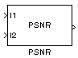# PSNR

Compute peak signal-to-noise ratio (PSNR) between images

•Libraries:
Computer Vision Toolbox / Statistics

## Description

The PSNR block computes the peak signal-to-noise ratio, in decibels, between two images. This ratio is used as a quality measurement between the original and a compressed image. The higher the PSNR, the better the quality of the compressed, or reconstructed image.

The mean-square error (MSE) and the peak signal-to-noise ratio (PSNR) are used to compare image compression quality. The MSE represents the cumulative squared error between the compressed and the original image, whereas PSNR represents a measure of the peak error. The lower the value of MSE, the lower the error.

To compute the PSNR, the block first calculates the mean-squared error using the following equation:

`$MSE=\frac{\sum _{M,N}{\left[{I}_{1}\left(m,n\right)-{I}_{2}\left(m,n\right)\right]}^{2}}{M*N}$`

In the previous equation, M and N are the number of rows and columns in the input images. Then the block computes the PSNR using the following equation:

`$PSNR=10{\mathrm{log}}_{10}\left(\frac{{R}^{2}}{MSE}\right)$`

In the previous equation, R is the maximum fluctuation in the input image data type. For example, if the input image has a double-precision floating-point data type, then R is 1. If it has an 8-bit unsigned integer data type, R is 255, etc.

### Computing PSNR for Color Images

Different approaches exist for computing the PSNR of a color image. Because the human eye is most sensitive to luma information, you can compute the PSNR for color images by converting the image to a color space that separates the intensity (luma) channel, such as YCbCr. The Y (luma), in YCbCr represents a weighted average of R, G, and B. G is given the most weight, again because the human eye perceives it most easily. Compute the PSNR only on the luma channel.

## Ports

### Input

expand all

Input image, specified as scalar, vector, or matrix.

Data Types: `single` | `double` | `int8` | `int16` | `int32` | `int64` | `uint8` | `uint16` | `uint32` | `uint64` | `Boolean` | `fixed point`

Input image, specified as scalar, vector, or matrix.

Data Types: `single` | `double` | `int8` | `int16` | `int32` | `int64` | `uint8` | `uint16` | `uint32` | `uint64` | `Boolean` | `fixed point`

### Output

expand all

Peak signal-to-noise ratio between images, returned as a scalar.

#### Dependencies

If the input is a fixed-point or integer data type, the block output is double-precision floating point. Otherwise, the block input and output are the same data type.

Data Types: `double`

## Block Characteristics

 Data Types `double` | `fixed point` | `integer` | `single` Multidimensional Signals `no` Variable-Size Signals `yes`

## Version History

Introduced before R2006a